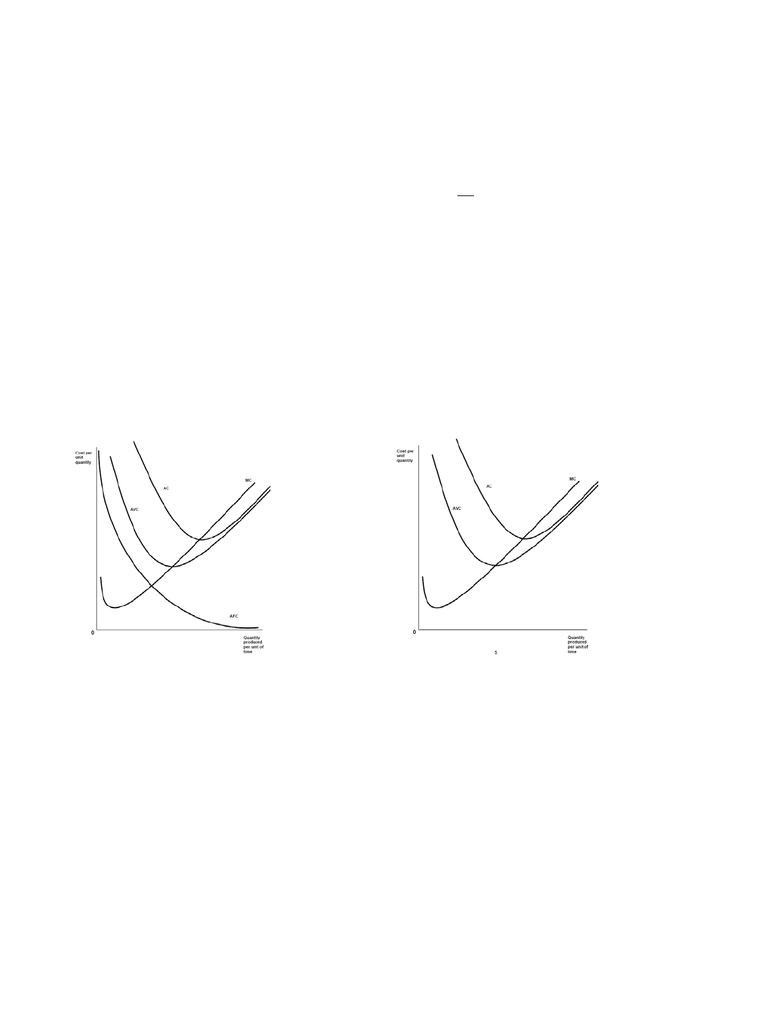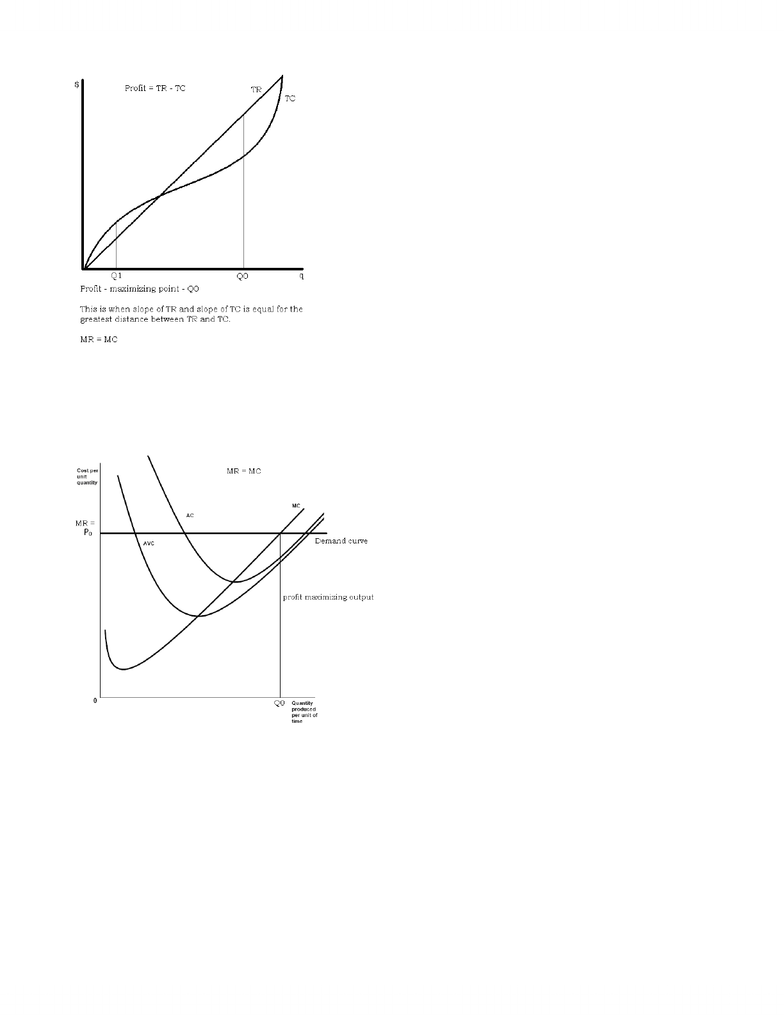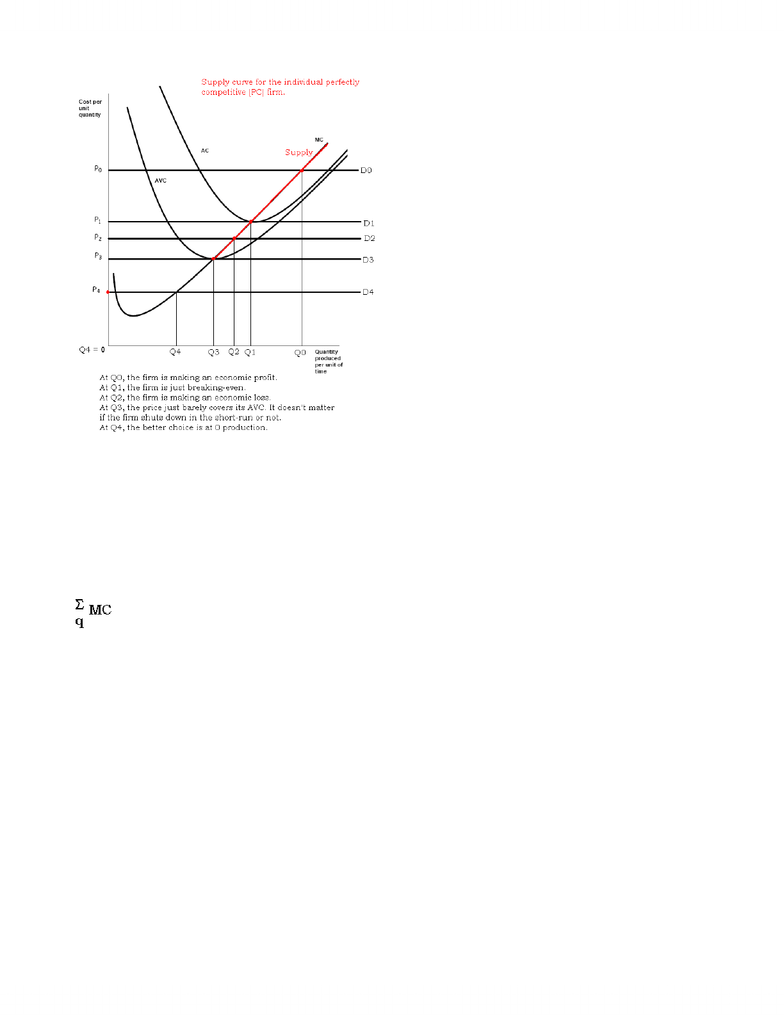# Lecture notes week 7

16 views8 pages
School
UTSC
Department
Economics for Management Studies
Course
MGEA02H3Week 7 – Perfect Competition
Perfect Competition – the main model for microeconomics
x the firm in SR
x the industry in SR
x the firm in LR
x the industry in LR
x dynamics of SR & LR adjustments to a “shock”—how does equilibrium change in SR & LR
x too much material to complete in one week, but included here because it is all one model
Perfect Competition
1. Many buyers, many sellers (price takers)
2. Homogeneous good…standardized (no brand loyalty)
3. Free entry and exit (no barriers to competition)
4. Perfect information (consumers do not make mistakes…always find the lowest price)
Typical cost situation facing perfectly competitive firm:
Rising MC in SR because of diminishing marginal product of labour (note that TC = (PL L) + (PK K), so that dTC/dL = PL
Also, we can write the marginal product of labour as MPL = dq/dL [where q = f(K, L)].
Therefore, the ratio PL/MPL = (dTC/dL)/(dq/dL) or dTC/dL dL/dq = dTC/dq
In other words: MC = PL/MPL!)
PL = price of labour
MPL = marginal product of labour
U-shaped AVC and AC curves
AFC declining as output increases
Look like diagram… Simplified a bit…
Remember, cost curves (and cost functions) in economics include all opportunity costs…including those that accountants don’t count (a
normal return on money invested in the firm, labour of family members and of the owner, etc.)
PC (perfectly competitive) firm will try to maximize profit. It can’t change its cost curves. It can’t push up the price. It can’t distinguish
its product from others by advertising/marketing/product innovation. All it can do is choose the profit-maximizing output!
3 = TR TC
Max q 3 = d TR / d q – d TC / d q = 0
i.e., MR – MC = 0 or MR = MC
i.e., choose the output at which MR = MC
www.notesolution.com
Unlock document

This preview shows pages 1-3 of the document.
Unlock all 8 pages and 3 million more documents.Because individual PC firm has no power over price, D curve is horizontal (perfectly elastic). PC firm is a “price taker”
TR = P x q
If P is a constant (to the firm), its marginal revenue is
MR = d TR / d q = d (P x q) /d q = P
MR = P for the perfectly competitive firm
Profit-maximizing supply decision by PC firm when P = P0
What happens to firm’s supply decision at different possible prices?
www.notesolution.com
Unlock document

This preview shows pages 1-3 of the document.
Unlock all 8 pages and 3 million more documents.Supply curve of individual PC firm is?
MC curve above min AVC curve
Why?
Breakeven price?
P = min AC
Shut-down price?
P = min AVC
Supply curve of PC industry in SR is?
Assuming all PC firms have identical costs
Supply curve of the industry
If MC = 2 + .1q
And there are 1000 identical firms in the PC industry
1000q = Q
or q = Q/1000 = 0.001Q
For firm, supply function is P = MC (above AVC, of course)
Industry supply function is P = MC with substitution for q
P = 2 + 0.1(0.001Q)
Or P = 2 + 0.0001Q (SR Industry Supply Curve)
Limitation on function: must be above min AVC
Algebraic example
Market Demand: P = 154 – 0.06Q
Total cost function of each PC firm: TC = 3q2 + 10q + 432 and FC = 384
So, TVC = 3q2 + 10q + 48
MC = d TC / d q = 6q + 10
AC = TC / q = 3q + 10 + 432 / q
AVC = TVC / q = 3q + 10 + 48 / q
Where does AC reach minimum?
d AC / d q = 3432 / q² = 0 or q = 12
AC at q = 12 is \$82
Where does AVC reach minimum?
www.notesolution.com
Unlock document

This preview shows pages 1-3 of the document.
Unlock all 8 pages and 3 million more documents.